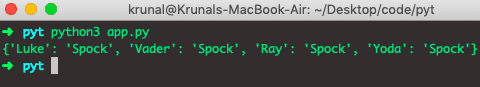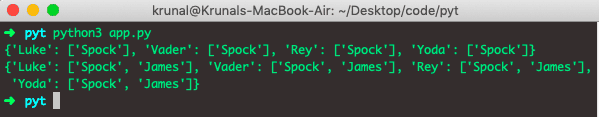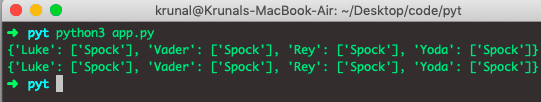# Python dict.fromkeys: The Complete Guide

0
577To create a dictionary from given keys in Python, use the dict.fromKeys() method.

## Python dict.fromkeys

The dict.fromKeys() is a built-in Python function that creates a new dictionary from the given sequence of elements with a value provided by the user. The fromKeys method returns the dictionary with specified keys and values.

The fromkeys() help us quickly achieve this task using a single method.

### Syntax

See the following syntax of Dictionary.fromKeys().

`dictionary.fromkeys(keys, value)`

### Arguments

The keys parameter is required, and it is an iterable specifying the keys of the new dictionary.

The value parameter is optional and the value for all keys. The default value is None.

### Return value

The dict.fromkeys() method returns a new dictionary with a given sequence of elements as the dictionary’s keys.

If a value argument is set, each element of the newly created dictionary is set to the provided value.

### Example

See the following example.

```# app.py

StarWars = ('Luke', 'Vader', 'Ray', 'Yoda')
StarTrek = ('Spock')
universe = dict.fromkeys(StarWars, StarTrek)
print(universe)```

See the output.So, we have created a dictionary using the fromKeys() method where we used StarWars as keys and StarTrek as value.

In the final output, the values will be the same for all the keys because the values will be mapped to each key. If you pass no value to the dictionary, then see the result. Check out the following code.

```# app.py

StarWars = ('Luke', 'Vader', 'Ray', 'Yoda')
universe = dict.fromkeys(StarWars)
print(universe)```

See the output.It will give us the value as a None for all the keys.

## Create a dictionary from Python List

We can create a dictionary from a Python List. See the following code.

```# app.py

StarWars = ['Luke', 'Vader', 'Ray', 'Yoda']
StarTrek = 'Spock'
universe = dict.fromkeys(StarWars, StarTrek)
print(universe)```

See the output.The fromKeys() can also be supplied with a mutable object as the default value. But in this case, a deep copy is made of a dictionary, i.e., if we append a value in the original list, the append takes place in all the values of keys.

If a provided value is the mutable object (whose value can be modified) like the list, dictionary, etc., when a mutable object is modified, each sequence element also gets updated.

Each element is assigned a reference to the same object (points to the same object in the memory).

See the following code.

```# app.py

StarWars = ['Luke', 'Vader', 'Rey', 'Yoda']
StarTrek = ['Spock']
universe = dict.fromkeys(StarWars, StarTrek)
print(universe)

StarTrek.append('James')
print(universe)```

See the output.The solution to the above problem is Dictionary Comprehension. See the following code.

```# app.py

StarWars = ['Luke', 'Vader', 'Rey', 'Yoda']
StarTrek = ['Spock']
universe = { key : list(StarTrek) for key in StarWars }
print(universe)

StarTrek.append('James')
print(universe)```

See the output.That’s it for this tutorial.

This site uses Akismet to reduce spam. Learn how your comment data is processed.